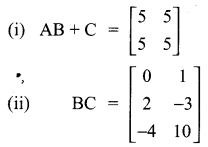# Samacheer Kalvi 10th Maths Solutions Chapter 3 Algebra Ex 3.19

## Tamilnadu Samacheer Kalvi 10th Maths Solutions Chapter 3 Algebra Ex 3.19

Multiple choice questions.
10th Maths Exercise 3.19 Answers Question 1.
A system of three linear equations in three variables is inconsistent if their planes
(1) intersect only at a point
(2) intersect in a line
(3) coincides with each other
(4) do not intersect.
Solution:
(4) do not intersect

10th Maths Exercise 3.19 Question 2.
The solution of the system x + y – 3z = – 6, -7y + 7z = 7, 3z = 9 is …………
(1) x = 1, y = 2, z = 3
(2) x = -1, y = 2, z = 3
(3) x = -1, y = -2, z = 3
(4) x = 1, y = 2, z = 3
(1) x = 1, y = 2, z = 3
Hint.
x + y – 3x = – 6 ….(1)
– 7y + 7z = 7 ….(2)
3z = 9 ….(3)
From (3) we get
z = $$\frac { 9 }{ 3 }$$ = 3
Substitute the value of z in (2)
-7y + 7(3) = 7
-7y = -14
Substitute the value of y = 2 and z = 3 in (1)
x + 2 – 3(3) = -6
x + 2 – 9 = -6
x = -6 + 7
x = 1
The value of x = 1, y = 2 and z = 3

Exercise 3.19 Class 10 Question 3.
If (x – 6) is the HCF of x2 – 2x – 24 and x2 – kx – 6 then the value of k is
(1) 3
(2) 5
(3) 6
(4) 8
Solution:
(2) 5

Ex 3.19 Class 10 Question 4.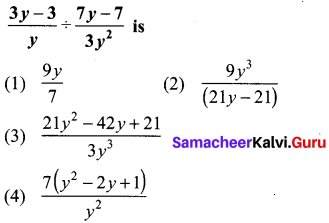Solution:
(1) $$\frac{9 y}{7}$$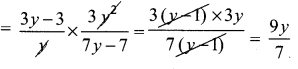10th Maths 3.19 Question 5.
$$\mathbf{y}^{2}+\frac{\mathbf{1}}{y^{2}}$$ is not equal to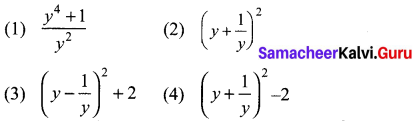Solution:
(2) $$\left(y+\frac{1}{y}\right)^{2}$$
Hint:
$$y^{2}+\frac{1}{y^{2}} \neq\left[y+\frac{1}{y}\right]^{2}$$

Samacheer Kalvi Guru 10th Maths Question 6.Solution:
(3) $$\frac{x^{2}-7 x+40}{(x+5)(x-5)(x+1)}$$
Hint: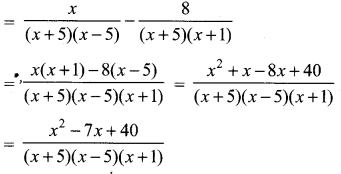Samacheer Kalvi 10th Maths Book Graph Solution Question 7.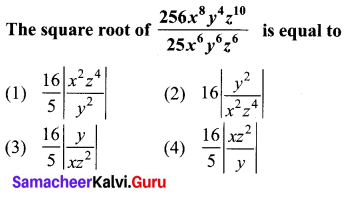Solution:
(4) $$\frac{16}{5}\left|\frac{x z^{2}}{y}\right|$$
Hint: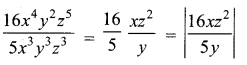Exercise 3.19 Question 8.
Which of the following should be added to make x4 + 64 a perfect square ……….
(1) 4x2
(2) 16x2
(3) 8x2
(4) -8x2
(2) 16x2
Hint.
x2 + 64 = (x2)2 + 82 – 2 × x2 × 8
= (x2 – 8)2
2 × x2 × 8 must be added

Samacheer Kalvi 10th Maths Book Graph Solutions Question 9.
The solution of (2x – 1)2 = 9 is equal to
(1) -1
(2) 2
(3) -1, 2
(4) None of these
Solution:
(3) -1, 2
Hint:
(2x – 1)2 = (±3)2
⇒ 2x – 1 = +3
2x – 1 = 3, 2x – 1 = – 3
2x = 4, 2x = – 2
x = 2,-1

Question 10.
The values of a and b if 4x4 – 24x3 + 76x2 + ax + b is a perfect square are
(1) 100, 120
(2) 10, 12
(3) -120, 100
(4) 12, 10
Solution:
(3) -120, 100
Hint: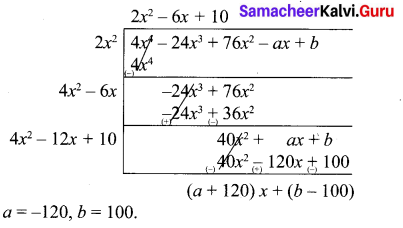Question 11.
If the roots of the equation q2x2 + p2x + r2 = 0 are the squares of the roots of the equation qx2 +px + r = 0, then q,p, r are in ______.
(1) A.P
(2) G.P
(3) Both A.P and G.P
(4) none of these
Solution:
(2) G.P
Hint: q2x2 + p2x + r2 = 0
(2) G.P.

Question 12.
Graph of a linear polynomial is a …………..
(1) straight line
(2) circle
(3) parabola
(4) hyperbola
(1) straight lineQuestion 13.
The number of points of intersection of the T quadratic polynomial x2 + 4x + 4 with the X axis.
(1) 0
(2) 1
(3) 0 or 1
(4) 2
Solution:
(2) 1
(x + 2)2 = (x + 2)(x + 2)
= x = -2, -2 = 1

Question 14.
For the given matrix A = $$\left[\begin{array}{cccc}{1} & {3} & {5} & {7} \\ {2} & {4} & {6} & {8} \\ {9} & {11} & {13} & {15}\end{array}\right]$$ the order of the matrix AT is
(1) 2 × 3
(2) 3 × 2
(3) 3 × 4
(4) 4 × 3
Solution:
(3) 3 × 4
Hint: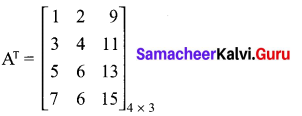Question 15.
If A is a 2 × 3 matrix and B is a 3 × 4 matrix, how many columns does AB have
(1) 3
(2) 4
(3) 2
(4) 5
Solution:
(2) 4
Hint: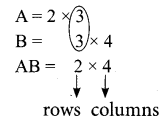Question 16.
If a number of columns and rows are not equal in a matrix then it is said to be a …………..
(1) diagonal matrix
(2) rectangular matrix
(3) square matrix
(4) identity matrix
(2) rectangular matrix

Question 17.
Transpose of a column matrix is
(1) unit matrix
(2) diagonal matrix
(3) column matrix
(4) row matrix
Solution:
(4) row matrix

Question 18.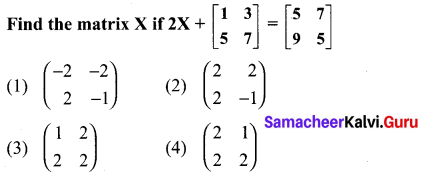Solution:
(2) $$\left(\begin{array}{cc}{2} & {2} \\ {2} & {-1}\end{array}\right)$$
Hint: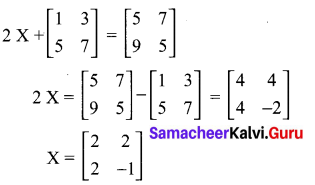Question 19.
Which of the following can be calculated from the given matrices
A = $$\left[\begin{array}{ll}{1} & {2} \\ {3} & {4} \\ {5} & {6}\end{array}\right]$$, B = $$\left[\begin{array}{lll}{1} & {2} & {3} \\ {4} & {5} & {6} \\ {7} & {8} & {9}\end{array}\right]$$
(i) A2
(ii) B2
(iii) AB
(iv) BA
(1) (i) and (ii) only
(2) (ii) and (iiii) only
(3) (ii) and (iv) only
(4) all of these
Solution:
(3) (ii) and (iv) only
Hint: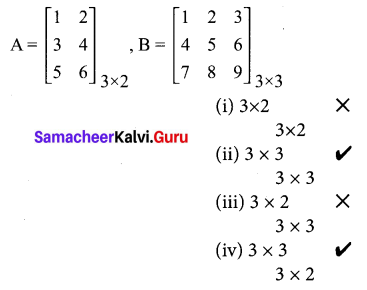Question 20.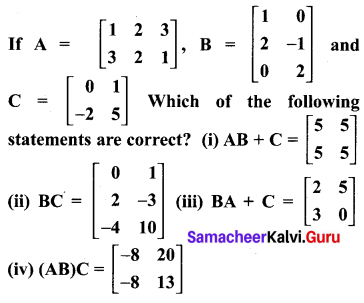(1) (i) and (ii) only
(2) (ii) and (iii) only
(3) (ii) and (iv) only
(4) all of these
Solution:
(1) (i) and (ii) only
Hint: